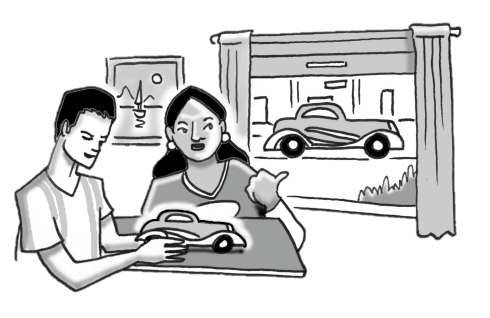### Home > CC3MN > Chapter 4 > Lesson 4.1.7 > Problem4-70

4-70.Josie and Jules are building a model car. They find that the real car is $54$ inches tall and $180$ inches long. They decide to make their model $3$ inches tall, but now they are having a disagreement. Josie thinks that their model should be $10$ inches long and Jules thinks it should be $129$ inches long. Help them settle their argument by deciding if either of them is correct. Explain how you know exactly how long their model should be.

Set up a proportion

$\frac{\text{car height}}{\text{car length}}=\frac{\text{ model height}}{\text{model length}}$

Substitute in the given information.

$\frac{54}{180}=\frac{3}{x}$

Multiply both sides by $x$ to get it in the numerator.

$x\left(\frac{54}{180}\right)=\left(\frac{3}{x}\right)x$

$\frac{54x}{180}=3$

Multiply both sides by $180$ to remove the fractions.

$180\left(\frac{54x}{180}\right)=3(180)$

Solve for $x$.

$54x=540$

Josie is correct.

$x=10$ inches